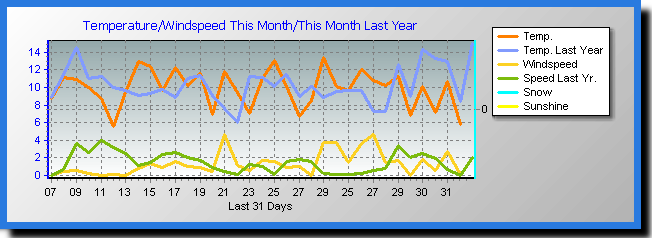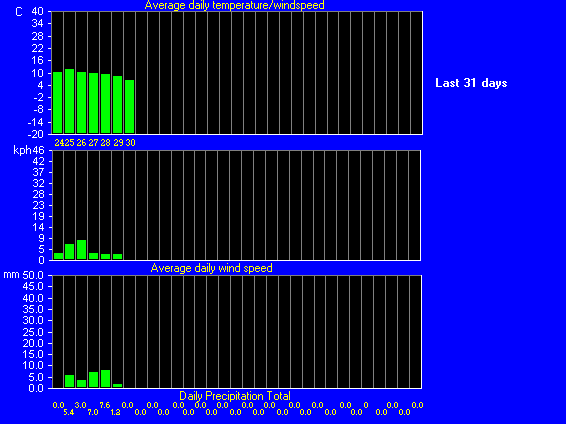Daily Report For July 2012#### Day Date

1
2
3
4
5
6
7
8
9
10
11
12
13
14
15
16
17
18
19
20
21
22
23
24
25
26
27
28
29
30
31

```
Averages\Extremes for day :25

------------------------------------------------------------

Average temperature     = 12.0°C

Average humidity        = 78%

Average dewpoint        = 8.1°C

Average barometer       = 1011.8 hPa

Average windspeed       = 6.7 km/h

Average gustspeed       = 11.7 km/h

Average direction       = 27° (NNE)

Rainfall for month      = 5.4 mm

Rainfall for year       = 384.6 mm

Rainfall for day        = 5.4 mm

Maximum rain per minute = 0.2 mm on day 25 at time 23:53

Maximum temperature     = 16.4°C on day 25 at time 12:34

Minimum temperature     = 8.3°C on day 25 at time 03:01

Maximum humidity        = 94% on day 25 at time 04:09

Minimum humidity        = 60% on day 25 at time 13:34

Maximum dewpoint        = 9.7°C on day 25 at time 23:25

Minimum dewpoint        = 7.0°C on day 25 at time 6:15

Maximum pressure        = 1018.0 hPa on day 25 at time 09:01

Minimum pressure        = 1007.9 hPa on day 25 at time 05:11

Maximum windspeed       = 22.2 km/h on day 25 at time 08:48

Maximum gust speed      = 35.2 km/h  from 00°( N ) on day 25 at time 08:44

Daily wind run          = 161.8km

Maximum heat index      = 16.4°C on day 25 at time 12:34

Averages\Extremes for day :26

------------------------------------------------------------

Average temperature     = 10.7°C

Average humidity        = 85%

Average dewpoint        = 8.3°C

Average barometer       = 1008.9 hPa

Average windspeed       = 9.0 km/h

Average gustspeed       = 14.5 km/h

Average direction       = 7° ( N )

Rainfall for month      = 8.4 mm

Rainfall for year       = 387.6 mm

Rainfall for day        = 3.0 mm

Maximum rain per minute = 0.2 mm on day 26 at time 08:36

Maximum temperature     = 13.2°C on day 26 at time 14:38

Minimum temperature     = 9.5°C on day 26 at time 06:08

Maximum humidity        = 95% on day 26 at time 09:00

Minimum humidity        = 72% on day 26 at time 14:45

Maximum dewpoint        = 9.2°C on day 26 at time 09:00

Minimum dewpoint        = 7.3°C on day 26 at time 9:23

Maximum pressure        = 1011.8 hPa on day 26 at time 09:00

Minimum pressure        = 1006.8 hPa on day 26 at time 14:52

Maximum windspeed       = 31.5 km/h on day 26 at time 13:56

Maximum gust speed      = 44.4 km/h  from 00°( N ) on day 26 at time 15:35

Daily wind run          = 216.8km

Maximum heat index      = 13.2°C on day 26 at time 14:38

Averages\Extremes for day :27

------------------------------------------------------------

Average temperature     = 10.3°C

Average humidity        = 90%

Average dewpoint        = 8.7°C

Average barometer       = 1016.4 hPa

Average windspeed       = 2.6 km/h

Average gustspeed       = 5.4 km/h

Average direction       = 227° ( SW)

Rainfall for month      = 15.4 mm

Rainfall for year       = 394.6 mm

Rainfall for day        = 7.0 mm

Maximum rain per minute = 0.4 mm on day 27 at time 09:19

Maximum temperature     = 14.8°C on day 27 at time 14:51

Minimum temperature     = 7.8°C on day 27 at time 04:21

Maximum humidity        = 99% on day 27 at time 08:52

Minimum humidity        = 77% on day 27 at time 15:03

Maximum dewpoint        = 11.5°C on day 27 at time 12:29

Minimum dewpoint        = 6.2°C on day 27 at time 2:11

Maximum pressure        = 1020.5 hPa on day 27 at time 08:57

Minimum pressure        = 1011.7 hPa on day 27 at time 09:06

Maximum windspeed       = 13.0 km/h on day 27 at time 20:54

Maximum gust speed      = 22.2 km/h  from 203°(SSW) on day 27 at time 20:54

Daily wind run          = 061.7km

Maximum heat index      = 14.8°C on day 27 at time 14:51

Averages\Extremes for day :28

------------------------------------------------------------

Average temperature     = 9.7°C

Average humidity        = 92%

Average dewpoint        = 8.4°C

Average barometer       = 1023.4 hPa

Average windspeed       = 1.9 km/h

Average gustspeed       = 4.2 km/h

Average direction       = 234° ( SW)

Rainfall for month      = 23.0 mm

Rainfall for year       = 402.2 mm

Rainfall for day        = 7.6 mm

Maximum rain per minute = 0.4 mm on day 28 at time 22:09

Maximum temperature     = 15.6°C on day 28 at time 14:12

Minimum temperature     = 6.3°C on day 28 at time 07:28

Maximum humidity        = 96% on day 28 at time 09:00

Minimum humidity        = 70% on day 28 at time 14:19

Maximum dewpoint        = 12.3°C on day 28 at time 12:54

Minimum dewpoint        = 5.5°C on day 28 at time 7:28

Maximum pressure        = 1027.8 hPa on day 28 at time 08:59

Minimum pressure        = 1019.1 hPa on day 28 at time 14:12

Maximum windspeed       = 18.5 km/h on day 28 at time 14:22

Maximum gust speed      = 31.5 km/h  from 225°( SW) on day 28 at time 16:13

Daily wind run          = 046.4km

Maximum heat index      = 15.6°C on day 28 at time 14:12

Averages\Extremes for day :29

------------------------------------------------------------

Average temperature     = 8.7°C

Average humidity        = 91%

Average dewpoint        = 7.2°C

Average barometer       = 1029.0 hPa

Average windspeed       = 2.1 km/h

Average gustspeed       = 4.5 km/h

Average direction       = 227° ( SW)

Rainfall for month      = 24.2 mm

Rainfall for year       = 403.4 mm

Rainfall for day        = 1.2 mm

Maximum rain per minute = 0.2 mm on day 29 at time 19:09

Maximum temperature     = 12.1°C on day 29 at time 14:55

Minimum temperature     = 5.2°C on day 29 at time 06:00

Maximum humidity        = 98% on day 29 at time 10:29

Minimum humidity        = 77% on day 29 at time 15:11

Maximum dewpoint        = 8.7°C on day 29 at time 11:52

Minimum dewpoint        = 4.4°C on day 29 at time 5:36

Maximum pressure        = 1031.5 hPa on day 29 at time 08:24

Minimum pressure        = 1027.0 hPa on day 29 at time 14:57

Maximum windspeed       = 14.8 km/h on day 29 at time 16:00

Maximum gust speed      = 24.1 km/h  from 225°( SW) on day 29 at time 13:06

Daily wind run          = 049.8km

Maximum heat index      = 12.1°C on day 29 at time 14:55

Averages\Extremes for day :30

------------------------------------------------------------

Average temperature     = 9.4°C

Average humidity        = 88%

Average dewpoint        = 7.4°C

Average barometer       = 1030.5 hPa

Average windspeed       = 3.3 km/h

Average gustspeed       = 6.3 km/h

Average direction       = 188° ( S )

Rainfall for month      = 24.2 mm

Rainfall for year       = 403.4 mm

Rainfall for day        = 0.0 mm

Maximum rain per minute = 0.0 mm on day 30 at time 09:00

Maximum temperature     = 13.7°C on day 30 at time 14:53

Minimum temperature     = 5.3°C on day 30 at time 08:04

Maximum humidity        = 97% on day 30 at time 09:35

Minimum humidity        = 66% on day 30 at time 14:51

Maximum dewpoint        = 9.7°C on day 30 at time 11:20

Minimum dewpoint        = 4.3°C on day 30 at time 7:59

Maximum pressure        = 1031.9 hPa on day 30 at time 10:17

Minimum pressure        = 1029.2 hPa on day 30 at time 05:14

Maximum windspeed       = 16.6 km/h on day 30 at time 15:02

Maximum gust speed      = 31.5 km/h  from 203°(SSW) on day 30 at time 16:13

Daily wind run          = 078.5km

Maximum heat index      = 13.7°C on day 30 at time 14:53

Averages\Extremes for day :31

------------------------------------------------------------

Average temperature     = 7.9°C

Average humidity        = 83%

Average dewpoint        = 5.0°C

Average barometer       = 1028.2 hPa

Average windspeed       = 2.1 km/h

Average gustspeed       = 3.9 km/h

Average direction       = 167° (SSE)

Rainfall for month      = 24.2 mm

Rainfall for year       = 403.4 mm

Rainfall for day        = 0.0 mm

Maximum rain per minute = 0.0 mm on day 31 at time 08:59

Maximum temperature     = 14.4°C on day 31 at time 13:18

Minimum temperature     = 2.7°C on day 31 at time 06:50

Maximum humidity        = 97% on day 31 at time 02:49

Minimum humidity        = 53% on day 31 at time 12:52

Maximum dewpoint        = 7.4°C on day 31 at time 10:17

Minimum dewpoint        = 1.8°C on day 31 at time 5:23

Maximum pressure        = 1031.2 hPa on day 31 at time 09:47

Minimum pressure        = 1026.8 hPa on day 31 at time 03:51

Maximum windspeed       = 16.6 km/h on day 31 at time 13:19

Maximum gust speed      = 22.2 km/h  from 135°( SE) on day 31 at time 14:14

Daily wind run          = 049.4km

Maximum heat index      = 14.4°C on day 31 at time 13:18

---------------------------------------------------------------------------------------------

Averages\Extremes for the month of July 2012

---------------------------------------------------------------------------------------------

Average temperature     = 9.9°C

Average humidity        = 86%

Average dewpoint        = 7.5°C

Average barometer       = 1021.3 hPa

Average windspeed       = 3.8 km/h

Average gustspeed       = 7.0 km/h

Average direction       = 0° ( N )

Rainfall for month      = 58.4 mm

Rainfall for year       = 437.6 mm

Maximum rain per minute = 0.4 mm on day 28 at time 22:09

Maximum temperature     = 16.4°C on day 25 at time 12:34

Minimum temperature     = 2.7°C on day 31 at time 06:50

Maximum humidity        = 99% on day 27 at time 08:52

Minimum humidity        = 53% on day 31 at time 12:52

Maximum dewpoint        = 12.3°C on day 28 at time 12:54

Minimum dewpoint        = 1.8°C on day 31 at time 5:23

Maximum pressure        = 1031.9 hPa on day 30 at time 10:17

Minimum pressure        = 1006.8 hPa on day 26 at time 14:52

Maximum windspeed       = 31.5 kmh from 00°( N ) on day 26 at time 13:56

Maximum gust speed      = 44.4 km/h from 00°( N ) on day 26 at time 15:35

Maximum heat index      = 16.4°C on day 25 at time 12:34

Avg daily max temp :14.4°C

Avg daily min temp :6.5°C

Growing degrees days :17.8 GDD

Total windrun = 714.1km

-----------------------------------

Daily rain totals

-----------------------------------

05.4 mm  on day 27

03.0 mm  on day 28

07.0 mm  on day 29

07.6 mm  on day 30

01.2 mm  on day 31

```